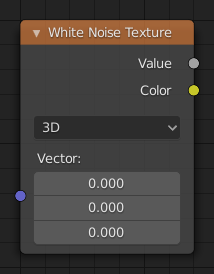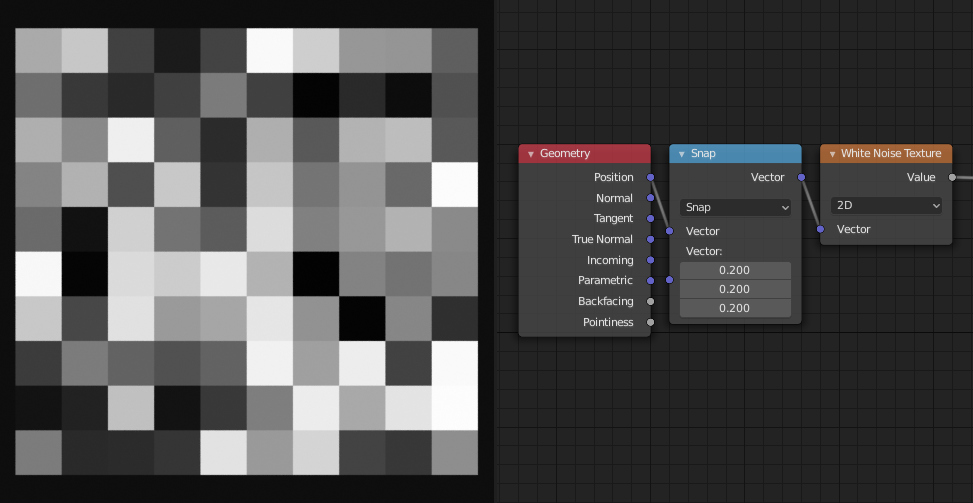# White Noise Texture Node¶

The White Noise Texture node returns a random number based on an input seed. The seed can be a number, a 2D vector, a 3D vector, or a 4D vector; depending on the Dimensions property. The output number ranges between zero and one.White Noise Texture Node.

## Inputs¶

The inputs are dynamic, they become available if needed depending on the node properties.

Vector

Vector used as seed in 2D, 3D, and 4D dimensions.

W

Value used as seed in 1D and 4D dimensions.

## Properties¶

Dimensions

The dimensions of the space to evaluate the noise in.

1D

The W input is used as seed.

2D

The X and Y components of the Vector input are used as seed.

3D

The Vector input is used as seed.

4D

Both the Vector input and the W input are used as seed.

## Outputs¶

Value

Output random value.

Color

Output random color.

## Examples¶Generating cell noise using the Snap vector operation and the White Noise node.